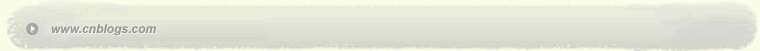Impossible is nothing 爱过知情重醉过知酒浓 　　花开花谢终是空 　　缘份不停留像春风来又走 　　女人如花花似梦

 < 2022年10月 >
2526272829301
2345678
9101112131415
16171819202122
23242526272829
303112345

• 随笔 - 8
• 文章 - 91
• 评论 - 16
• 引用 - 0

# 相册

•

### 评论排行榜

1.什么是STL？

2.为什么要使用类属算法？

template <typename T>

T max(T x,T y)

{

if(x<y) ruturn y;

else return x;

}

int u=3,v=4;

double d=4.7;

cout<<max(u,v)<<endl;

cout<<max(d,9.3)<<endl;

cout<<max(u,d)<<endl; //incorrect

3.类属算法如何工作？

4.基本概念

template<typename T1,typename T2>

class pair{

public:

T1 first;

T2 second;

pair():first(T1()),sencond(T2()){}   //默认构造函数

pair(const T1&  x,const T2&  y):first(x),second(y){}  //自定义构造函数

} ;

pair<int,char> pair1(13,'a');

pair<bool,double> pair2(true,0.1);

template <typename T>

class vector{

//.....

public:

template <typename InputIterator>

void insert(iterator position,InputIterator first,InputIterator last);

//.....

};

vector<T> 提供对变长序列的随机访问，对序列末尾的插入和删除操作时间是分摊常量的，对序列开头的插入和删除是线性的。可以理解为封装后的数组。

depue<T>提供对变长序列的随机访问，对序列开头和末尾的插入和删除操作是分摊常量的。存储空间是连续的（这一点尚未在书上说明，只是个人推测）。

list<T>提供对变长序列的线性时间访问，但是对序列中任意位置的插入和删除操作均为常量时间的。可以理解为封装后的链表。

#include<iostream>
#include<vector>
#include<cassert>
#include<string>
#include<algorithm> //for reverse
using namespace std;

template<typename Container>
Container make(const char s[])
{
return Container(&s,&s[strlen(s)]);
}

int main()
{
cout<<"Using reverse algorithm with a vector"<<endl;
string string1="mark twain";
vector<char> vector1(string1.begin(),string1.end()); //begin()和end()是访问器，返回迭代器
reverse(vector1.begin(),vector1.end());
assert(vector1==make< vector<char> >("niawt kram"));
cout<<" --OK."<<endl;
return 0;
}

set<Key>支持唯一的键（每个键值只能有一个）并提供对见本身的快速检索。

multiset<Key>支持客重复的键（同一个键值可以有多个副本）并提供对键本身的快速检索。

map<Key,T>支持唯一的（Key类型的）键并提供对另一个基于键的类型T的快速检索 。

multimap<Key,T>支持可重复的（Key类型的）键并提供对另一个基于键的类型T的快速检索。

#include<iostream>
#include<map>
#include<string>
using namespace std;

int main()
{
map<string,long> directory;
directory["Bogart"]=123;
directory["Bacall"]=456;
directory["Cagney"]=789;
string name;
while(cin>>name)
{
if(directory.find(name)!=directory.end())
cout<<"The phone number for "<<name
<<" is "<<directory[name]<<"\n";
else
cout<<"Sorry,no listing for "<<name<<endl;
}
return 0;
}

STL容器和其他C++容器类库中的容器的一个重要区别：STL容易并没有为其所包含的容器提供过多的操作（成员函数），相反，STL提供的是类属算法。

#include<iostream>
#include<cassert>
#include<list>
#include<algorithm>
using namespace std;

template<typename Container>
Container make(const char s[])
{
return Container(&s,&s[strlen(s)]);
}

int main()
{
list<char> list1=make< list<char> >("C++ is a better C.");
list<char>::iterator
where=find(list1.begin(),list1.end(),'e');
list<char>::iterator next=where;
++next;
assert(*where=='e' && *next=='t');
cout<<" ---OK."<<endl;
return 0;
}

merge算法的功能是将两个序列的元素合并到一个序列中，调用形式为

merge(first1,last1,first2,last2,result);

*迭代器first1和last1表示一个输入序列的起始和终止位置，其元素类型为T;

*迭代器first2和last2表示另一个输入序列的起始和终止位置，其元素类型也为T;

*按照类型T上的<运算符的定义，两个输入序列均为升序排列。

*result表示合并后序列存放的起始位置。

merge函数返回的结果是合并两个序列后的一个升序序列。

#include<iostream>
#include<string>
#include<cassert>
#include<vector>
#include<list>
#include<algorithm>
using namespace std;

template<typename Container>
Container make(const char s[])
{
return Container(&s,&s[strlen(s)]);
}

int main()
{
char s[]="acegikm";
vector<char> vector1(
make< vector<char> >("bdfhjlnopqrstuvwxyz"));
list<char> list1(26,'x'); //将list1初始化为26个'x'

//merge first 5 letters in array s with first 10 in
//vector1,putting result in list1
merge(&s,&s,vector1.begin(),vector1.begin()+10,list1.begin());

assert(list1==
make< list<char> >("abcdefghijlnopqxxxxxxxxxxx"));
cout<<" --OK."<<endl;
return 0;
}

deque<char> deque1(
make< deque<char> >("bdfhjlnopqrstuvwxyz"));

1. ==   !=  *

2.   ++

3.     --

4.+=  -=  +  -  <  >  <=  >=

#include<iostream>
#include<vector>
#include<cassert>
#include<numeric>  //for accumulate
using namespace std;

int main()
{
int x={2,3,5,7,11};
vector<int> vector1(&x,&x);
int sum=accumulate(vector1.begin(),vector1.end(),0); //最后一个参数是累加的初始值
assert(sum==28);
cout<<"--OK."<<endl;
return 0;
}

template <typename InputIterator,typename T,typename BinaryOperation>
T accumulate(InputIterator first,InputIterator last,
T init,BinaryOperation binary_op)
{
while (first!=last)
{
init=binary_op(init,*first);
++first;
}
return init;
}

#include<iostream>
#include<vector>
#include<cassert>
#include<numeric> //for accumulate
using namespace std;

int mult(int x,int y){return x*y;}

int main()
{
int x={2,3,5,7,11};
vector<int> vector1(&x,&x);
int product=
accumulate(vector1.begin(),vector1.end(),1,mult);
assert(product==2310);
cout<<"--OK."<<endl;
return 0;
}

#include<iostream>
#include<vector>
#include<cassert>
#include<numeric>  //for accumulate
using namespace std;

class multiply
{
public:
int operator()(int x,int y) const{return x*y;}
};

int main()
{
int x={2,3,5,7,11};
vector<int> vector1(&x,&x);
int product=
accumulate(vector1.begin(),vector1.end(),1,multiply());
assert(product==2310);
cout<<"--OK."<<endl;
return 0;
}

reverse_iterator将某种类型的迭代器变成一种新的迭代器，但保持其功能不变，而仅将其便利的顺序倒转过来。

#include<iostream>
#include<vector>
#include<cassert>
#include<numeric> //for accumulate
using namespace std;

int main()
{
float small=(float)1.0/(1<<26);
float x={1.0,3*small,2*small,small,small};
vector<float> vector1(&x,&x);
cout<<"Values to be added: "<<endl;
vector<float>::iterator i;
for(i=vector1.begin(); i != vector1.end(); ++i)
cout<<*i<<endl;
cout<<endl;

float sum=accumulate(vector1.begin(),vector1.end(),(float)0.0);
cout<<"Sum accumulated from left = "<<sum<<endl;

float sum1=accumulate(vector1.rbegin(),vector1.rend(),(float)0.0);
cout<<"sum accumulated from right = "<<(double)sum1<<endl;

return 0;
}

vector<float>::reverse_iterator类型是用迭代器适配器定义的。也可以在程序中直接使用适配器：

reverse_iterator<vector<float>::iterator> start(vector1.end()),finish(vector1.begin());

float sum1=accumulate(start,finish,(float)0.0);

reverse_iterator类型也提供了++和--运算符，但互换了这两个运算符的含义。上例中的vector1.rbegin()和vector1.rend()成员函数返回的即是reverse_iterator类型。

posted on 2006-02-22 22:04 笑笑生 阅读(768) 评论(1)  编辑 收藏 引用 所属分类: C++语言只有注册用户登录后才能发表评论。 【推荐】超50万行VC++源码: 大型组态工控、电力仿真CAD与GIS源码库 相关文章:Copyright © 笑笑生 Powered by: 博客园 模板提供：沪江博客